# LightGBM Tuner: New Optuna Integration for Hyperparameter Optimization

In this article, we will introduce the LightGBM Tuner in Optuna, a hyperparameter optimization framework, particularly designed for machine learning.

The LightGBM Tuner is one of Optuna’s integration modules for optimizing hyperparameters of LightGBM. The usage of LightGBM Tuner is straightforward. You use LightGBM Tuner by changing one import statement in your Python code. Using expert heuristics, LightGBM Tuner enables you to tune hyperparameters in less time than before. In the second half of this article, we confirm this with benchmarks.

# Naive method for tuning hyperparameters on LightGBM

LightGBM is a popular library that provides a fast, high-performance gradient boosting framework based on decision tree algorithms. While various features are implemented, it contains many hyperparameters to be tuned. Some examples are the hyperparameter that controls the number of leaves of a decision tree, the proportion of features to be sampled for each decision tree, and the minimum number of samples allocated to leaves of a decision tree. Tuning them manually requires many trials. Optuna is a framework designed to efficiently find better hyperparameters.

When tuning the hyperparameters of LightGBM using Optuna, a naive example code could look as follows:

In this example, Optuna tries to find the best combination of seven different hyperparameters, such as `feature_fraction`, `num_leaves`. The total number of combinations is a product of all the hyperparameter search spaces, resulting in a huge search space as depicted below.

# Step-wise algorithm

A major challenge in hyperparameter optimization is getting better evaluation with as few trials as possible. In particular, machine learning tasks typically require long computation time for a single trial, so it’s preferable to reduce the number of trials.

While there are many search methods that try to achieve better evaluation with few trials, let us think about search space. Among experts who have manually tuned the hyperparameters of LightGBM, there is a well-used and efficient method, named stepwise algorithm. It tunes the important hyperparameter variables in order.

In the above method, the hyperparameter variables are determined sequentially. This results in a compact search space, a sum of all spaces, as depicted below.

LightGBM Tuner is a module that implements the stepwise algorithm.

# Usage of LightGBM Tuner

LightGBM Tuner was released as an experimental feature in Optuna v0.18.0. You can try it by changing the import statement as follows:

Full example code is available in our repository.

Hyperparameter tuning starts when you call `lgb.train()` in your Python code. The “best parameters” and “search history” from the results of tuning can be obtained by passing Python objects as keyword arguments to `lgb.train()`.

In the current implementation, `lgb.train()` function is the only supported LightGBM API.

# Benchmarks

Can LightGBM Tuner achieve better performance than the naive tuning method? To answer this question, we benchmarked the performance of hyperparameter search methods using several popular datasets. The datasets used are shown in the table below.

In this benchmark, we selected three methods for comparison.

• [Tuner] Tuning with Step-wise algorithm by LightGBM Tuner
• [TPE] TPE (Tree-structured Parzen Estimator) + Naive tuning
• [Random] Random sampling + Naive tuning

TPE is one of the efficient search methods using stochastic models, and is the default sampling algorithm used in Optuna. In this benchmark, as in , by evaluating the trade-offs between the evaluation score and the number of trials, we compared the performance of three search methods.

To eliminate the effects of randomness, we performed a total of 20 experiments on each dataset and averaged the evaluation score by trial, as depicted below.

The following figure summarizes the results of the benchmark.

The red cross markers show the result when using LightGBM’s default parameters without any tuning. The horizontal axes represent the number of trials, and the vertical axes represent the best evaluation result in previous trials. AUC (Area under the ROC Curve) is used as an evaluation metric, and a higher value indicates a better result.

In this benchmark, we found that LightGBM Tuner achieved better evaluation results on 6 of the 9 datasets.

# Quantitative analysis: Examining the key hyperparameters

As shown in the benchmark result, LightGBM Tuner can outperform the other methods. We will dig deeper into the behavior of LightGBM using real data and consider how to improve the tuning algorithm more.

LightGBM Tuner selects a single variable of hyperparameter to tune step by step. For example, `feature_fraction`, `num_leaves`, and so on respectively. Which of these hyperparameters was important to tune for the optimization process in our benchmark result? The following violin plots show the results of the change in validation score when tuning each variable.

The larger the width, the greater the effect in the evaluation value. This indicates that the effect of tuning the variable is significant. As you can see in the above figure, depending on the dataset, the most important variable is different. In our benchmark, the most important variable was one of `feature_fraction`, `num_leaves` or `bagging_fraction`. The current implementation of LightGBM Tuner fixes the order of the tuning variables and the number of trials for each variable. By searching for hyperparameters that have a large effect and tuning them in the ideal order, further improvements may be possible.

# Conclusion

We introduced LightGBM Tuner, a new integration module in Optuna to efficiently tune hyperparameters and experimentally benchmarked its performance. In addition, by analyzing the experimental results, we confirmed that the important hyperparameters vary in different datasets, and discussed further improvements in the tuning algorithm.

An advantage of LightGBM Tuner is that you can try it with just one line change without thinking about any hyperparameters. Experimental results show that it can be a powerful tool, especially for new LightGBM users.

# Reference

•  Jean-François Puget. “Beyond Feature Engineering and HPO“, presented at Kaggle Days Paris
•  門脇大祐, 坂田隆司, 保坂桂佑, 平松雄司. “Kaggleで勝つデータ分析の技術”, 技術評論社
•  James Bergstra, Rémi Bardenet, Yoshua Bengio, Balázs Kégl, “Algorithms for Hyper-Parameter Optimization”, In Proc. of the International Conference on Advances in Neural Information Processing Systems (NIPS’11).
•  Stefan Falkner, Aaron Klein, Frank Hutter, “BOHB: Robust and Efficient Hyperparameter Optimization at Scale”, In Proc. of the 35th International Conference on Machine Learning (ICML’18).

--

--

--

## More from Optuna

A hyperparameter optimization framework

## Approximating the Minds of 2019 Oscars Voters using Neural Networks## Machine Learning Talks I am Excited about at mlcon 2.0## How Machine Learning and AI Can Improve Travel Services## OpenAI’s GPT — Part 1: Unveiling the GPT Model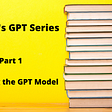## NLP Made Easy With These Libraries## How to evaluate the performance of a classifier## Neural Style Transfer in Tensorflow 2.0## Serve Recommendation Models with TorchServe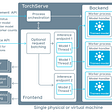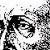## How to distribute hyperparameter tuning using Ray Tune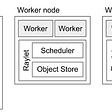## How to Speed Up XGBoost Model Training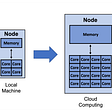## How I used sklearn’s LabelEncoder function to solve Kaggles February 2022 tabular competition## 100x Times CatBoost Speedup for Large Data Sets